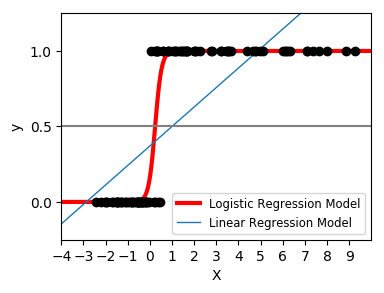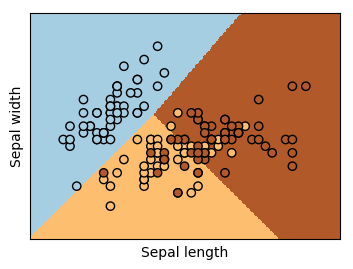# 机器学习系列1：算法基础-Logistic回归## logistic分布f(x)f(x)与F(x)F(x)图像如下，其中分布函数是以(μ,12)(μ,12)为中心对阵，γγ越小曲线变化越快。## logistic回归模型### 参数估计

P(Y=1|x)=π(x),P(Y=0|x)=1−π(x)P(Y=1|x)=π(x),P(Y=0|x)=1−π(x)## 概论

logistic_regression_path类则比较特殊，它拟合数据后，不能直接来做预测，只能为拟合数据选择合适逻辑回归的系数和正则化系数。主要是用在模型选择的时候。一般情况用不到这个类，所以后面不再讲述logistic_regression_path类。

``sklearn.linear_model.LogisticRegression(penalty='l2', dual=False, tol=0.0001, C=1.0, fit_intercept=True, intercept_scaling=1, class_weight=None, random_state=None, solver='liblinear', max_iter=100, multi_class='ovr', verbose=0, warm_start=False, n_jobs=1)``
``sklearn.linear_model.LogisticRegressionCV(Cs=10, fit_intercept=True, cv=None, dual=False, penalty='l2', scoring=None, solver='lbfgs', tol=0.0001, max_iter=100, class_weight=None, n_jobs=1, verbose=0, refit=True, intercept_scaling=1.0, multi_class='ovr', random_state=None)``
``logistic_regression_path(X, y, pos_class=None, Cs=10, fit_intercept=True, max_iter=100, tol=0.0001, verbose=0, solver='lbfgs', coef=None, copy=False, class_weight=None, dual=False, penalty='l2', intercept_scaling=1.0, multi_class='ovr', random_state=None, check_input=True, max_squared_sum=None, sample_weight=None)``

## 正则化选择参数：penalty

LogisticRegression和LogisticRegressionCV默认就带了正则化项。penalty参数可选择的值为”l1″和”l2″.分别对应L1的正则化和L2的正则化，默认是L2的正则化。

penalty参数的选择会影响我们损失函数优化算法的选择。即参数solver的选择，如果是L2正则化，那么4种可选的算法{‘newton-cg’, ‘lbfgs’, ‘liblinear’, ‘sag’}都可以选择。但是如果penalty是L1正则化的话，就只能选择‘liblinear’了。这是因为L1正则化的损失函数不是连续可导的，而{‘newton-cg’, ‘lbfgs’,‘sag’}这三种优化算法时都需要损失函数的一阶或者二阶连续导数。而‘liblinear’并没有这个依赖。

## 优化算法选择参数：solver

solver参数决定了我们对逻辑回归损失函数的优化方法，有4种算法可以选择，分别是：
a) liblinear：使用了开源的liblinear库实现，内部使用了坐标轴下降法来迭代优化损失函数。
b) lbfgs：拟牛顿法的一种，利用损失函数二阶导数矩阵即海森矩阵来迭代优化损失函数。
c) newton-cg：也是牛顿法家族的一种，利用损失函数二阶导数矩阵即海森矩阵来迭代优化损失函数。
d) sag：即随机平均梯度下降，是梯度下降法的变种，和普通梯度下降法的区别是每次迭代仅仅用一部分的样本来计算梯度，适合于样本数据多的时候，SAG是一种线性收敛算法，这个速度远比SGD快。关于SAG的理解，参考博文线性收敛的随机优化算法之 SAG、SVRG（随机梯度下降）

## 分类方式选择参数：multi_class

multi_class参数决定了我们分类方式的选择，有 ovr和multinomial两个值可以选择，默认是 ovr。
ovr即前面提到的one-vs-rest(OvR)，而multinomial即前面提到的many-vs-many(MvM)。如果是二元逻辑回归，ovr和multinomial并没有任何区别，区别主要在多元逻辑回归上。
OvR的思想很简单，无论你是多少元逻辑回归，我们都可以看做二元逻辑回归。具体做法是，对于第K类的分类决策，我们把所有第K类的样本作为正例，除了第K类样本以外的所有样本都作为负例，然后在上面做二元逻辑回归，得到第K类的分类模型。其他类的分类模型获得以此类推。

## 类型权重参数： class_weight

class_weight参数用于标示分类模型中各种类型的权重，可以不输入，即不考虑权重，或者说所有类型的权重一样。如果选择输入的话，可以选择balanced让类库自己计算类型权重，或者我们自己输入各个类型的权重，比如对于0,1的二元模型，我们可以定义class_weight={0:0.9, 1:0.1}，这样类型0的权重为90%，而类型1的权重为10%。

sklearn的官方文档中，当class_weight为balanced时，类权重计算方法如下：
n_samples / (n_classes * np.bincount(y))，n_samples为样本数，n_classes为类别数量，np.bincount(y)会输出每个类的样本数，例如y=[1,0,0,1,1],则np.bincount(y)=[2,3]

## 实例（线性回归和Logistic回归对比）

In :
```import numpy as np
import matplotlib.pyplot as plt

from sklearn import linear_model

# this is our test set, it's just a straight line with some
# Gaussian noise
xmin, xmax = -5, 5
n_samples = 100
np.random.seed(0)
X = np.random.normal(size=n_samples)
y = (X > 0).astype(np.float)
X[X > 0] *= 4
X += .3 * np.random.normal(size=n_samples)

X = X[:, np.newaxis]
# run the classifier
clf = linear_model.LogisticRegression(C=1e5)
clf.fit(X, y)

# and plot the result
plt.figure(1, figsize=(4, 3))
plt.clf()
plt.scatter(X.ravel(), y, color='black', zorder=20)
X_test = np.linspace(-5, 10, 300)

def model(x):
return 1 / (1 + np.exp(-x))
loss = model(X_test * clf.coef_ + clf.intercept_).ravel()
plt.plot(X_test, loss, color='red', linewidth=3)

ols = linear_model.LinearRegression()
ols.fit(X, y)
plt.plot(X_test, ols.coef_ * X_test + ols.intercept_, linewidth=1)
plt.axhline(.5, color='.5')

plt.ylabel('y')
plt.xlabel('X')
plt.xticks(range(-5, 10))
plt.yticks([0, 0.5, 1])
plt.ylim(-.25, 1.25)
plt.xlim(-4, 10)
plt.legend(('Logistic Regression Model', 'Linear Regression Model'),
loc="lower right", fontsize='small')
plt.show()
```## 小运用，多分类情况

In :
```import numpy as np
import pandas as pd
import matplotlib.pyplot as plt
from sklearn import linear_model, datasets
import numpy as np
```
In :
```# 导入数据，还是我们经典的iris
```
Out:
sepal length in cmsepal width in cmpetal length in cmpetal width in cmclass
05.13.51.40.2Iris-setosa
14.93.01.40.2Iris-setosa
24.73.21.30.2Iris-setosa
34.63.11.50.2Iris-setosa
45.03.61.40.2Iris-setosa
In :
```X = np.array(iris.iloc[:, :2])
Y = np.array(iris['class'])
Y[Y == 'Iris-setosa'] = 0
Y[Y == 'Iris-versicolor'] = 1
Y[Y == 'Iris-virginica'] = 2
Y = np.array(Y,dtype = np.int64)
Y
```
Out:
```array([0, 0, 0, 0, 0, 0, 0, 0, 0, 0, 0, 0, 0, 0, 0, 0, 0, 0, 0, 0, 0, 0, 0,
0, 0, 0, 0, 0, 0, 0, 0, 0, 0, 0, 0, 0, 0, 0, 0, 0, 0, 0, 0, 0, 0, 0,
0, 0, 0, 0, 1, 1, 1, 1, 1, 1, 1, 1, 1, 1, 1, 1, 1, 1, 1, 1, 1, 1, 1,
1, 1, 1, 1, 1, 1, 1, 1, 1, 1, 1, 1, 1, 1, 1, 1, 1, 1, 1, 1, 1, 1, 1,
1, 1, 1, 1, 1, 1, 1, 1, 2, 2, 2, 2, 2, 2, 2, 2, 2, 2, 2, 2, 2, 2, 2,
2, 2, 2, 2, 2, 2, 2, 2, 2, 2, 2, 2, 2, 2, 2, 2, 2, 2, 2, 2, 2, 2, 2,
2, 2, 2, 2, 2, 2, 2, 2, 2, 2, 2, 2])```
In :
```h = .02  # step size in the mesh

logreg = linear_model.LogisticRegression(C=1e5)

# we create an instance of Neighbours Classifier and fit the data.
logreg.fit(X, Y)
```
Out:
```LogisticRegression(C=100000.0, class_weight=None, dual=False,
fit_intercept=True, intercept_scaling=1, max_iter=100,
multi_class='ovr', n_jobs=1, penalty='l2', random_state=None,
solver='liblinear', tol=0.0001, verbose=0, warm_start=False)```
In :
```x_min, x_max = X[:, 0].min() - .5, X[:, 0].max() + .5
y_min, y_max = X[:, 1].min() - .5, X[:, 1].max() + .5
xx, yy = np.meshgrid(np.arange(x_min, x_max, h), np.arange(y_min, y_max, h))
Z = logreg.predict(np.c_[xx.ravel(), yy.ravel()])
```
In :
```# Put the result into a color plot
Z = Z.reshape(xx.shape)
plt.figure(1, figsize=(4, 3))
plt.pcolormesh(xx, yy, Z, cmap=plt.cm.Paired)

# Plot also the training points
plt.scatter(X[:, 0], X[:, 1], c=Y, edgecolors='k', cmap=plt.cm.Paired)
plt.xlabel('Sepal length')
plt.ylabel('Sepal width')

plt.xlim(xx.min(), xx.max())
plt.ylim(yy.min(), yy.max())
plt.xticks(())
plt.yticks(())

plt.show()
```2. 机器学习系列2：算法基础-K-Means
3. 机器学习系列3：算法基础-决策树
4. 机器学习系列4：进阶算法-SVM
5. 机器学习系列5：进阶算法-随机森林
6. 数学教学系列6：ARMA模型
7. 数学教学系列7：拉格朗日对偶问题
8. 数学教学系列8：梯度下降法
9. 数学教学系列9：B-S期权定价模型